# Electric Magnetic Fields Electric and magnetic fields manifest

• Slides: 26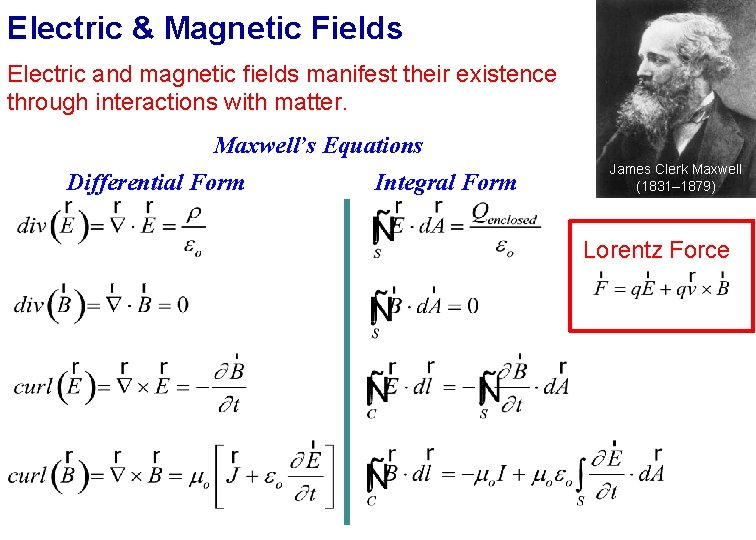Electric & Magnetic Fields Electric and magnetic fields manifest their existence through interactions with matter. Maxwell’s Equations Differential Form Integral Form James Clerk Maxwell (1831– 1879) Lorentz ForceLecture 1 Electric Charges & Coulomb’s Law http: //www. diyhappy. com/wpcontent/images/Lightning. bmp http: //andreacarlisle. files. wordp ress. com/2012/02/staticcling_dogs. jpg www. ehow. com/how_2180464_%20 reducestatic-cling. htmlElectric Charge Electric charge is an intrinsic characteristic of the fundamental particles that make up objects. Positive Charge Negative Charge + • Electrically neutral: object contains equal amounts of positive and negative charges • Net charge: imbalance in chargeElectric Charge Net charge of a system: algebraic sum of all the charges Law: Conservation of charge The net charge of a closed system never changesElectric Charge Electric charge is quantized \$=n http: //scrapetv. com/News%20 Pages/B usiness/images/us%20 penny. jpg • Elementary charge: e = 1. 60602176462(63) x 10– 19 C Coulomb (C): one coulomb is the amount of charge that is transferred through the cross section of a wire in 1 second when there is a current of 1 ampere in the wire.Charge of Particles Charge Electron Positron Proton Anti-Proton Neutron 0 Photon 0 Up Quark Down Quark Nucleus charge= +Ze, atom with Z electrons is neutral. Proton charge: |e+ | = 1. 60 x 10– 19 C Electron charge: |e- | = 1. 60 x 10– 19 CInteraction of Charges Charged objects interact by exerting forces on one another. � DEMO: Rod & FurConductors versus Insulators • Conductors: material in which electric charges can move around “freely. • Insulators: material in which electric charges are “frozen” in place. • Semi-conductor: material in which electric charges can move around but not as freely as in conductors. • Super-conductor: no resistance to the movement of charge.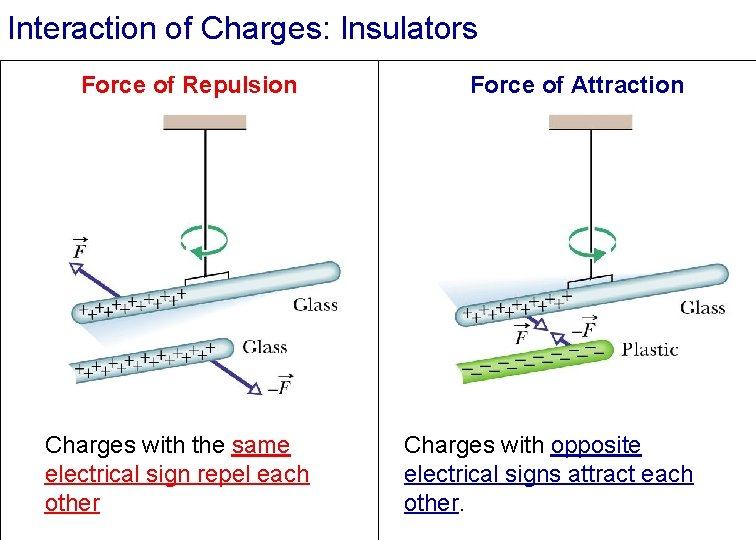Interaction of Charges: Insulators Force of Repulsion Charges with the same electrical sign repel each other Force of Attraction Charges with opposite electrical signs attract each other.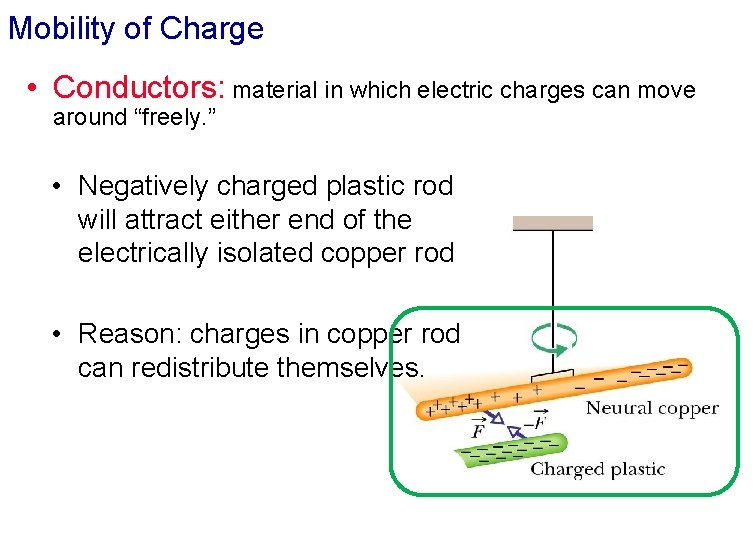Mobility of Charge • Conductors: material in which electric charges can move around “freely. ” • Negatively charged plastic rod will attract either end of the electrically isolated copper rod • Reason: charges in copper rod can redistribute themselves.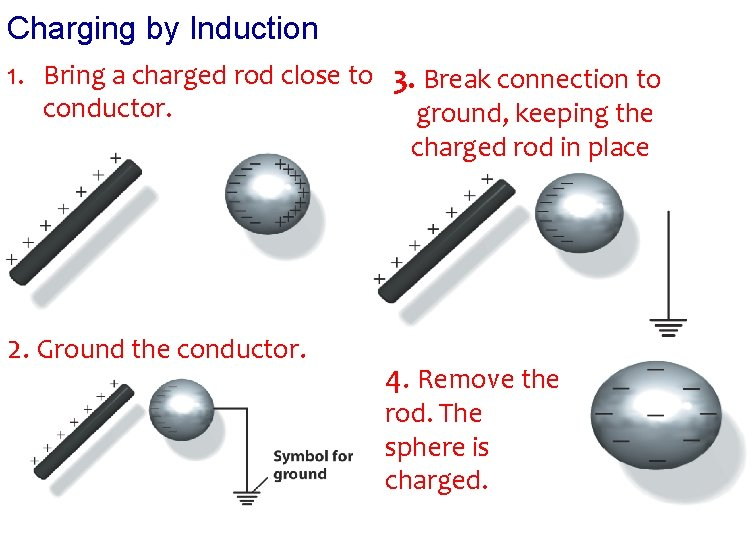Charging by Induction 1. Bring a charged rod close to 3. Break connection to conductor. ground, keeping the charged rod in place 2. Ground the conductor. 4. Remove the rod. The sphere is charged.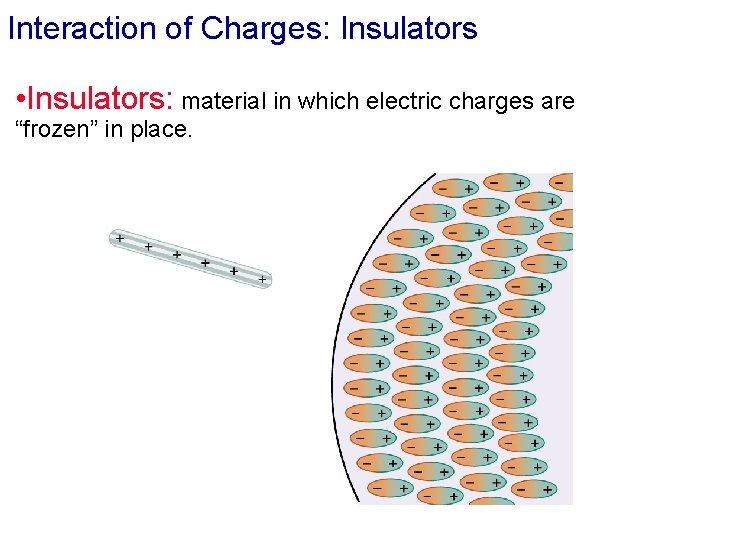Interaction of Charges: Insulators • Insulators: material in which electric charges are “frozen” in place.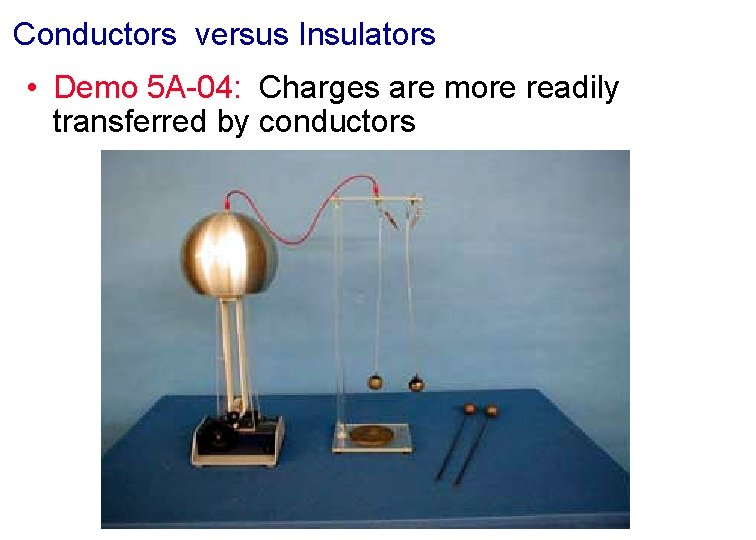Conductors versus Insulators • Demo 5 A-04: Charges are more readily transferred by conductorsMobility of Charge • Demo: Pie Tins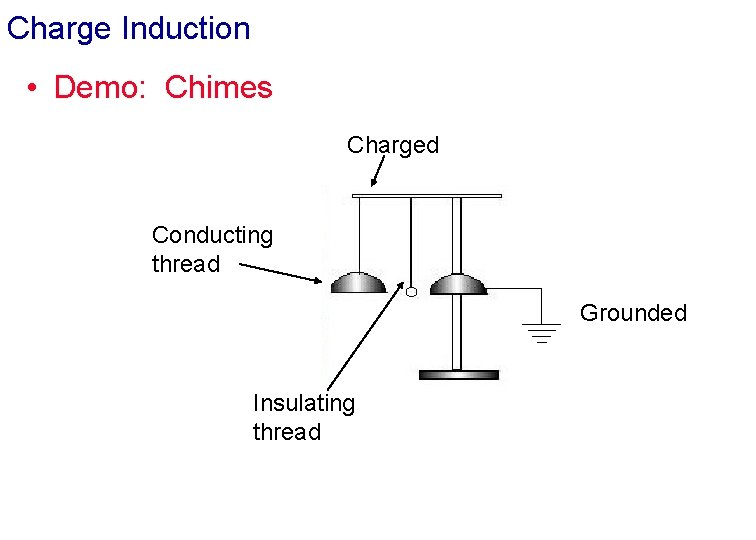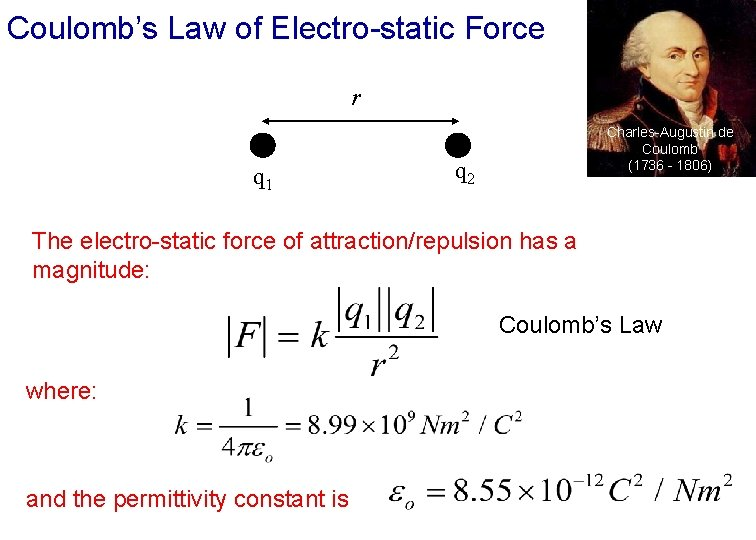Coulomb’s Law of Electro-static Force r q 1 Charles-Augustin de Coulomb (1736 - 1806) q 2 The electro-static force of attraction/repulsion has a magnitude: Coulomb’s Law where: and the permittivity constant is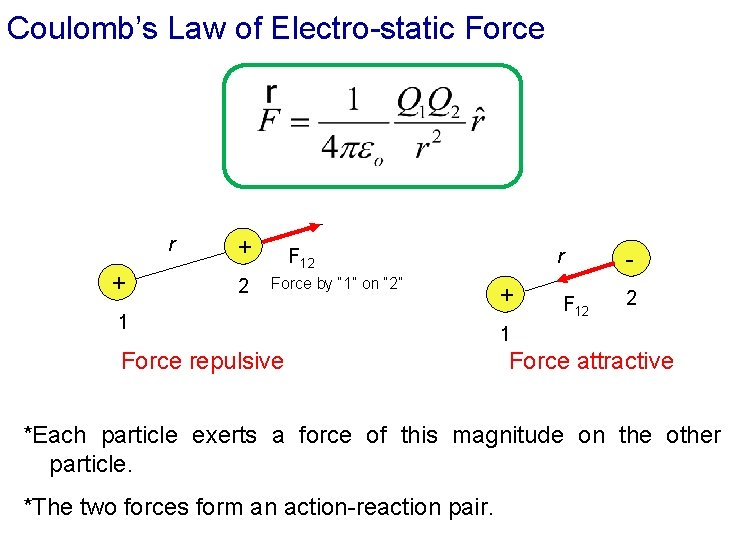Coulomb’s Law of Electro-static Force r + + 2 F 12 Force by “ 1” on “ 2” 1 Force repulsive r + F 12 2 1 Force attractive *Each particle exerts a force of this magnitude on the other particle. *The two forces form an action-reaction pair.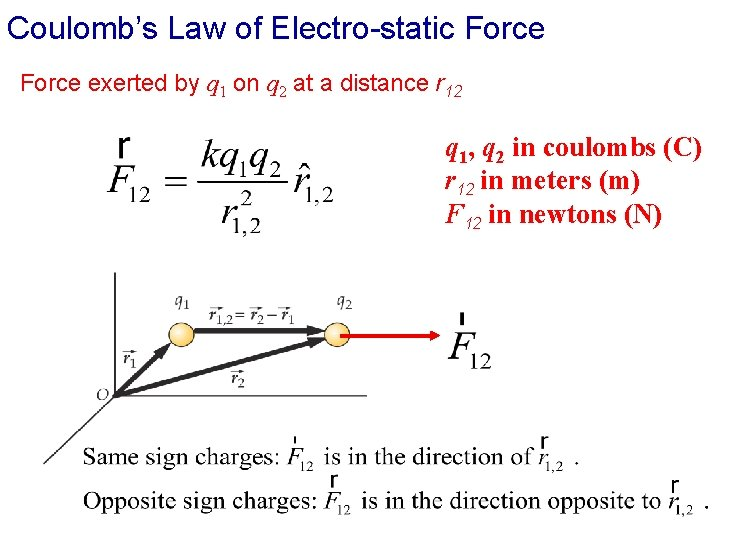Coulomb’s Law of Electro-static Force exerted by q 1 on q 2 at a distance r 12 q 1, q 2 in coulombs (C) r 12 in meters (m) F 12 in newtons (N)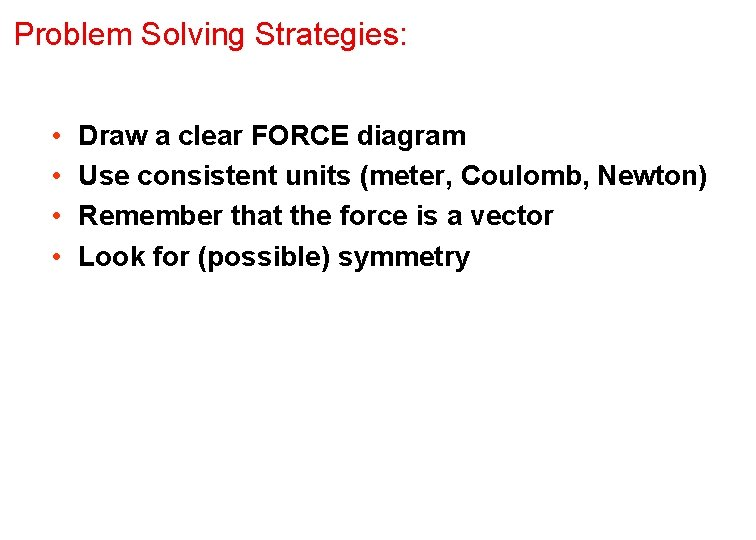Problem Solving Strategies: • • Draw a clear FORCE diagram Use consistent units (meter, Coulomb, Newton) Remember that the force is a vector Look for (possible) symmetryQuiz 1. Two charges q = + 1 µC and Q = +10 µC are placed near each other as shown below. Which diagram best depicts the electrostatic forces acting on the charges? +1 µC A B C +10 µC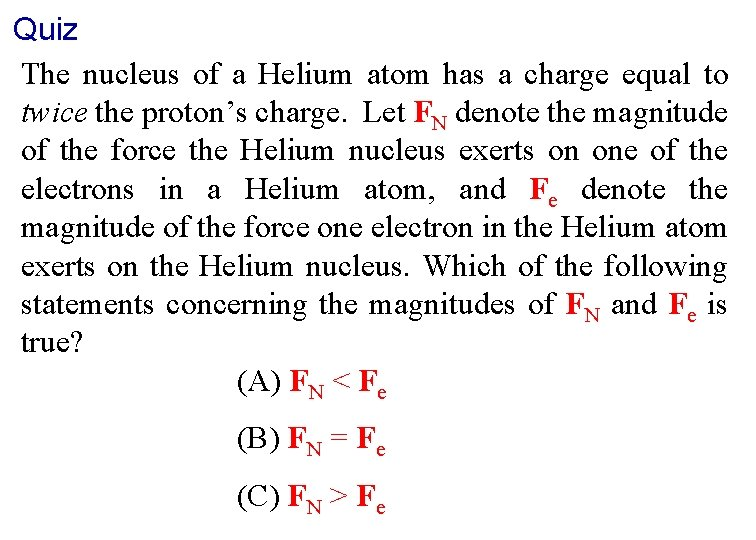Quiz The nucleus of a Helium atom has a charge equal to twice the proton’s charge. Let FN denote the magnitude of the force the Helium nucleus exerts on one of the electrons in a Helium atom, and Fe denote the magnitude of the force one electron in the Helium atom exerts on the Helium nucleus. Which of the following statements concerning the magnitudes of FN and Fe is true? (A) FN < Fe (B) FN = Fe (C) FN > Fe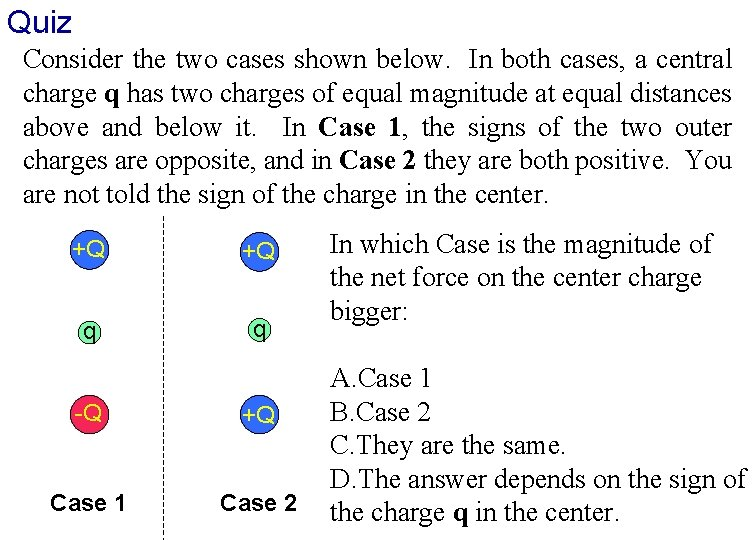Quiz Consider the two cases shown below. In both cases, a central charge q has two charges of equal magnitude at equal distances above and below it. In Case 1, the signs of the two outer charges are opposite, and in Case 2 they are both positive. You are not told the sign of the charge in the center. +Q +Q q q -Q +Q Case 1 Case 2 In which Case is the magnitude of the net force on the center charge bigger: A. Case 1 B. Case 2 C. They are the same. D. The answer depends on the sign of the charge q in the center.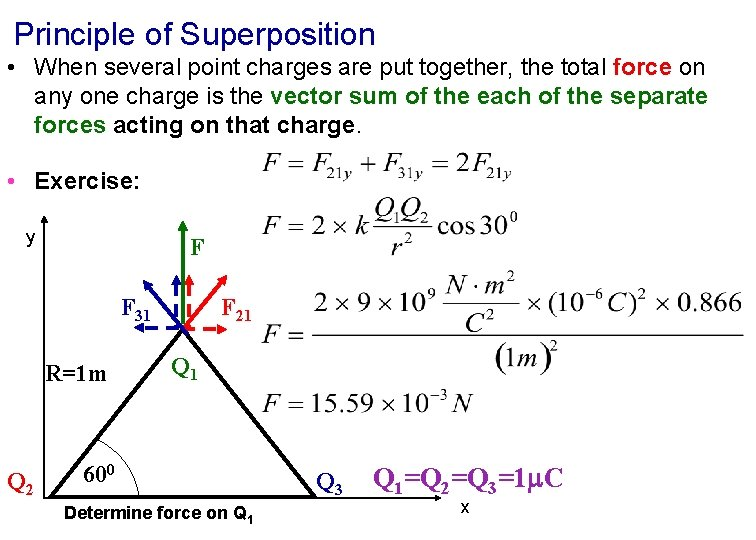Principle of Superposition • When several point charges are put together, the total force on any one charge is the vector sum of the each of the separate forces acting on that charge. • Exercise: y F F 31 R=1 m Q 2 F 21 Q 1 600 Determine force on Q 1 Q 3 Q 1=Q 2=Q 3=1 C xCoulomb’s Law Analogous to Newton’s Equation of Gravitation Analogous * k electro-static constant * Inverse Square Law * Charge * G gravitational constant * Inverse Square Law * Mass DIFFERS *Attractive/repulsive depending on sign of charges *Two kinds of charges *Dominates on small scale *Always attractive *One kind of mass *Dominates on large scalesElectro-Static Force versus Newton’s Force of Gravitational Attraction l Fel between the proton and the electron in a hydrogen atom in the ground state. From the Bohr model r=0. 53 x 10 -10 m. l Given such strong electrical interactions, atoms tend to remain uncharged. Matter prefers to be neutral. l Forces we experience, if not gravitational, are electrical in nature (even though the net charge may be zero).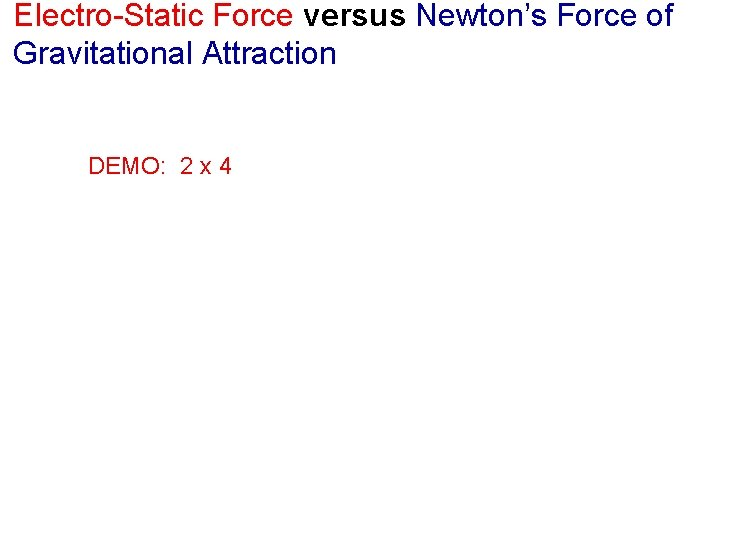Electro-Static Force versus Newton’s Force of Gravitational Attraction DEMO: 2 x 4